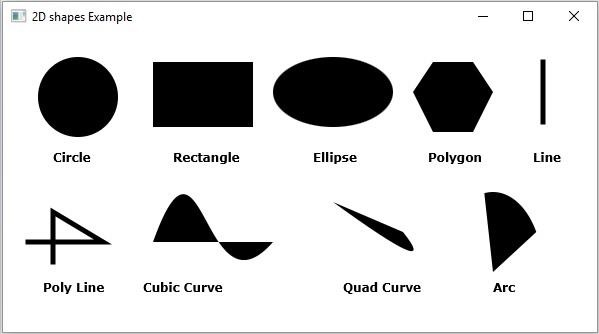# What are various 2D shapes provided by JavaFX?

Following are various geometrical shapes that you can draw using JavaFX

• Line − A line is a geometrical structure joining two-point. The javafx.scene.shape.The line class represents a line in the XY plane.

• Rectangle − A rectangle is a four-sided polygon that has two pairs of parallel and concurrent sides with all interior angles as right angles. The javafx.scene.The Rectangle class represents a rectangle in the XY plane.

• Circle − A circle is a line forming a closed loop, every point on which is a fixed distance from a center point. The javafx.scene.The Circle class represents a circle in the XY plane.

• Ellipse − An ellipse is defined by two points, each called a focus. If any point on the ellipse is taken, the sum of the distances to the focus points is constant. The javafx.scene.Ellipse's class represents an ellipse in the XY plane.

• Polygon − A closed shape formed by a number of coplanar line segments connected end to end is known as a polygon. The javafx.scene.Polygon class represents a polygon in the XY plane.

• Polyline − A polyline is the same as a polygon except that a polyline is not closed in the end. Or, a continuous line composed of one or more line segments. The javafx.scene.Polyline class represents a polyline in the XY plane.

• Cubic Curve − A cubic curve is a Bezier parametric curve in the XY plane is a curve of degree 3. The javafx.scene.CubicCurve class represents a cubic curve in the XY plane.

• QuadCurve − A quadratic curve is a Bezier parametric curve in the XY plane is a curve of degree 2. The javafx.scene.QuadCurve class represents a quad curve in the XY plane.

• Arc − An arc is part of a curve. The javafx.scene.Arc class represents an arc in the XY plane.

To create a required shape you need to −

• Instantiate the respective class.

• Set its properties.

• Add the created object to Group.

## Example

Following JavaFX Example demonstrates the creation of all the available 2D shapes −

import javafx.application.Application;
import javafx.scene.Group;
import javafx.scene.Scene;
import javafx.stage.Stage;
import javafx.scene.shape.Arc;
import javafx.scene.shape.ArcType;
import javafx.scene.shape.Circle;
import javafx.scene.shape.CubicCurve;
import javafx.scene.shape.Ellipse;
import javafx.scene.shape.Line;
import javafx.scene.shape.Polygon;
import javafx.scene.shape.Polyline;
import javafx.scene.shape.Rectangle;
import javafx.scene.text.Font;
import javafx.scene.text.FontPosture;
import javafx.scene.text.FontWeight;
import javafx.scene.text.Text;
public class JavaFXShapes extends Application {
public void start(Stage stage) {
Font font = Font.font("verdana", FontWeight.BOLD, FontPosture.REGULAR, 12);
Text cirText = new Text("Circle");
cirText.setFont(font);
cirText.setX(50);
cirText.setY(130);
Text rectText = new Text("Rectangle");
rectText.setFont(font);
rectText.setX(170);
rectText.setY(130);
Text ellipseText = new Text("Ellipse");
ellipseText.setFont(font);
ellipseText.setX(310);
ellipseText.setY(130);
Text polyText = new Text("Polygon");
polyText.setFont(font);
polyText.setX(425);
polyText.setY(130);
Text lineText = new Text("Line");
lineText.setFont(font);
lineText.setX(530);
lineText.setY(130);
Text polyLineText = new Text("Poly Line");
polyLineText.setFont(font);
polyLineText.setX(40);
polyLineText.setY(260);
Text cubicCurveText = new Text("Cubic Curve");
cubicCurveText.setFont(font);
cubicCurveText.setX(140);
cubicCurveText.setY(260);
Text arcText = new Text("Arc");
arcText.setFont(font);
arcText.setX(490);
arcText.setY(260);
//Drawing a circle
Circle circle = new Circle(75.0f, 65.0f, 40.0f );
//Drawing a Rectangle
Rectangle rect = new Rectangle(150, 30, 100, 65);
//Drawing an ellipse
Ellipse ellipse = new Ellipse(330, 60, 60, 35);
//Drawing Polygon
Polygon poly = new Polygon(410, 60, 430, 30, 470, 30, 490, 60, 470, 100, 430, 100 );
//Drawing a Line
Line line = new Line(540, 30, 540, 90);
line.setStrokeWidth(5.0);
//Drawing a Poly line
Polyline polyLine = new Polyline(25, 210, 100, 210, 50, 180, 50, 230);
polyLine.setStrokeWidth(5.0);
//Drawing a cubic curve
CubicCurve cubicCurve = new CubicCurve(150.0, 210.0, 200.0, 70.0, 200.0, 290.0, 270.0, 210.0);
//Drawing an arc
Arc arc = new Arc(490, 240, 50, 80, 30, 70);
arc.setType(ArcType.ROUND);
//Setting the stage
Group root = new Group(
circle, ellipse, rect, poly, line,
cirText, rectText, ellipseText, polyText, lineText,
Scene scene = new Scene(root, 600, 300);
stage.setTitle("2D shapes Example");
stage.setScene(scene);
stage.show();
}
public static void main(String args[]){
launch(args);
}
}

## Output